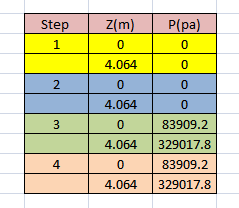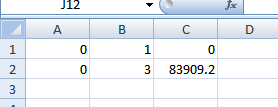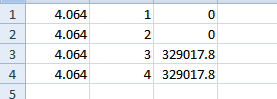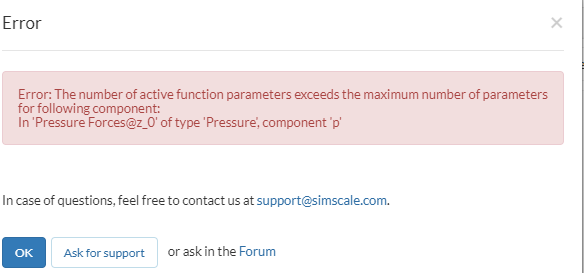# Analyzing a bolted assembly of SATURN V

Hey,
Trying to do analysis of SATURN V’s bolted nozzle assembly for assignment
The problem mentioned is:

“The pressure due to the exhaust gas in the nozzle is calculated using 1D gas dynamics. It is assumed to vary linearly along the nozzle axis. The pressure at the exit (z=0) is 12.17 psi and the pressure at the entrance to the mid-nozzle is 47.72 psi.
The regeneration channels are omitted in the model. In exchange, a free body diagram is used to deduce the equivalent forces on the mid nozzle and lower nozzle (the upper nozzle is not modeled here). This force pair is modeled as two separate forces, each of 1000 lbf. The gas temperature is 700 F which causes thermal strain. We assume that bolt is pre-loaded to 50% of its breaking strength.”

For example: If I wanted to do this simulation in four steps. I want to provide preloaded tension on the bolt for all four steps and pressure which is varying linearly in 3rd and 4th not in 1st and 2nd.
So how can I provide the Pressure condition on two value of z top and bottom and meanwhile switching them on at the 3rd and 4th step?

3 Likes

Hi @ojagtap,

The link below discusses how to turn loads on or off using either a table or formula for time based simulations. I think this is what you are inquiring about in your last paragraph. This same method can also be used to vary loads based on position.

Please let me know if this helps out or if you have additional questions.

Thanks,
Christopher

4 Likes

Thanks for the suggestion
As done by MR. Ahmed Hussain. He has mentioned two pressures i.e. Pressure1 and Pressure 2 at a single time step so now how can I define that Pressure 1 is for upper position @ z=0.0 m and pressure 2 @ z=4.064 m for lower of the nozzle. Couldn’t see his project as it has been removed.
Wanted to provide condition as:Hi @ojagtap!

I have updated the link to one of the project that gives the example of using formulas in displacement. Considering your example, you can apply different pressure at different interval using either formula or table upload. Your case seems quite similar to one of my project here: Thermal Shock Analysis of a Globe Valve

In the above project, pretension in the bolts are applied using temperature difference that occurs in the very first step of the simulation i.e. the temperature is dropped instantly from 373.15 K (100 oC) to 293.15 K (20 oC) in the first iteration of the simulation. This is one of the technique for prestressing. If you want to apply prestressing using physical contacts, please have a look at this project here: Finite Element Analysis of Prestressing of a Bolt

Just have a look and let me know if you run in to any problem.

Best,
Ahmed

3 Likes

Thanks for sharing the projects, sir.
Got a lot to learn from your projects. also had a look at your Thermomechanical Analysis of Shrink Fitting of Tool Holder that one helped.
Actually, I had confusion in these areas

1. I wanted to provide Frictionless support (Used in Ansys), Normal displacement = 0, Tangential traction = 0. As the Cad model has only middle and lower nozzle upper nozzle is not mentioned.
Thought of using Fixed value constrain with normal displacement =0.

2. And I thought of providing pressure varying with temperature and z, with two different boundary condition

1st one for z=0:(Column 1,2,3) representing (cloumn(z,t,P) respectively2nd one for z=4,064But facing errorI am not sure how to exactly implement it in SimScale but I think you can use elastic support under constraints to do so. In that you can use orthogonal method through which you can constraint the structure with a high elasticity of the spring in certain direction while allowing movement in others.

As I have explained before also. If you want to utilize 2 to 3 varying quantities, then you should move to the formula rather than table. Because table upload only works with 2 quantities. So either you can have z in first column and pressure in second or time in first column and pressure in second, not all of them together. I would recommend to first play with time only and apply two different pressures to two different surfaces at different time span.

For info. on elastic support, please see here: Elastic support - documentation

and validation: Free vibrations on elastic support

Hope this helps.

Best,
Ahmed

2 Likes

Just some info. on Frictional support of Ansys: http://inside.mines.edu/~apetrell/ENME442/Labs/1301_ENME442_lab2.1.pdf

May be someone can help here better to solve this caseBest,
Ahmed

1 Like

Hi,
frictionless support with a tangential traction of 0 in ANSYS is equivalent to symmetry constraint on SimScale. Both fix the displacement in the direction normal to the surface and allow it in tangential directions. If traction !=0 is added an orthotropic elastic support could be used to model this, as @ahmedhussain18 already suggested.

If the surface where the frictionless support should be applied, is orthogonal to any of the coordinate directions, I would advice to use an equivalent fixed value constraint instead of “symmetry”. It reduces the risk of creating an over-constrained system, as explained here: Pin - the problem with contacts

Best,
Richard

3 Likes